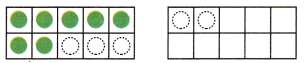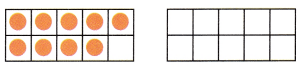All the solutions provided in McGraw Hill Math Grade 1 Answer Key PDF Chapter 7 Lesson 5 Adding by Making 10 as per the latest syllabus guidelines.

Solve

Question 1.
7 + 5 = ?10 + 2 = 12
so 7 + 5 = 12
Explanation:
In a ten frame there are 7 green dots showing the number 7
to make ten we need 3 more in first ten frame and 2 more in second ten frame.

Question 2.
9 + 7 = ?10 + 6 = ___
so 9 + 7 = ___